PSABM4ZE - Practice---Acc7.3 Lesson 7 Reasoning about Solving Equations (Part 1) (7.EE.B.4.a)

Explain how the parts of the balanced hanger compare to the parts of the equation.

23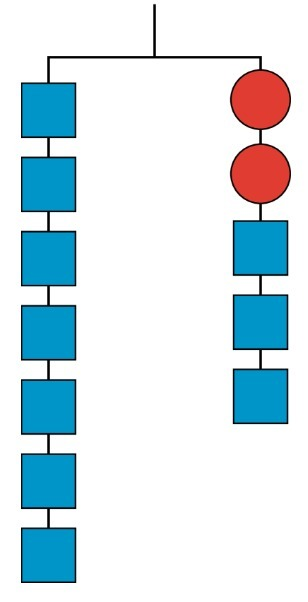Part A)

Here is a hanger: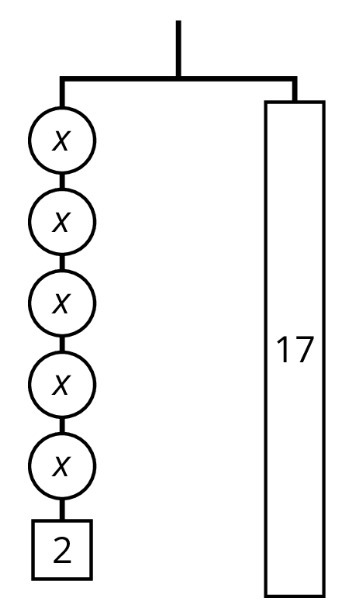Write an equation to represent the hanger.

Write the equation using the "WIRIS editor" button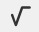.

Part B)

Draw more hangers to show each step you would take to find x. Explain your reasoning.

Copy the diagram of the hanger above and show the steps on paper, take a picture, and upload it using the image upload icon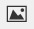.

If you do not have the ability to upload an image of your work type "Steps are on paper."

Part C)

Write an equation to describe each hanger you drew.

Write the equation using the "WIRIS editor" button.

Part D)

Describe how each equation matches its hanger.

Part E)

What does x equal?

Type your answer below as a number (example: 5, 3.1, 4 1/2, or 3/2):
Part A)

Clare drew this diagram to match the equation 2x + 16 = 50, but she got the wrong solution as a result of using this diagram.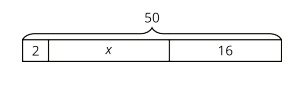Copied for free from Illustrative Mathematics

What value for x can be found using the diagram?

Type your answer below as a number (example: 5, 3.1, 4 1/2, or 3/2):
Part B)

Clare drew this diagram to match the equation 2x + 16 = 50, but she got the wrong solution as a result of using this diagram.Show or explain how to fix Clare’s diagram to correctly match the equation.

Take a picture of your new diagram and upload it using the insert image icon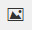. If you are not able to take a picture, explain how you could fix Clare's diagram.